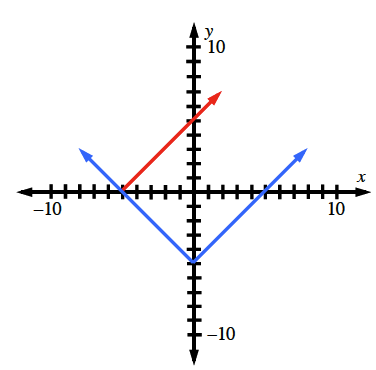Home > CCA2 > Chapter 7 > Lesson 7.1.3 > Problem7-42

7-42.

Solve $| x + 5 | = | x | - 5$ by graphing. Express your solution algebraically. Homework Help ✎The solution is the value of x that makes both of these equations true. Where do the graphs overlap?

x ≤ −5